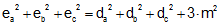Problem 254. Triangle, Centroid, Vertices, any Point, Distances, Squares The figure shows a triangle ABC. G is the centroid G (point of concurrency of the triangle's medians) and E is any point (interior/exterior). If AG = da, BG = db, CG = dc, AE = ea, BE = eb, CE = ec, and EG = m, prove that. View or post a solution.Home | Sitemap | Geometry | Problems | 251-260 | View or post a solution